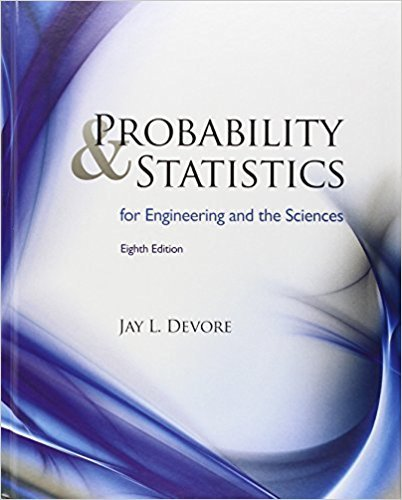×
×

# Solutions for Chapter 15: Distribution-Free Procedures## Full solutions for Probability and Statistics for Engineering and the Sciences | 8th Edition

ISBN: 9780538733526Solutions for Chapter 15: Distribution-Free Procedures

Solutions for Chapter 15
4 5 0 269 Reviews
31
5
##### ISBN: 9780538733526

Chapter 15: Distribution-Free Procedures includes 36 full step-by-step solutions. This textbook survival guide was created for the textbook: Probability and Statistics for Engineering and the Sciences , edition: 8. This expansive textbook survival guide covers the following chapters and their solutions. Since 36 problems in chapter 15: Distribution-Free Procedures have been answered, more than 26619 students have viewed full step-by-step solutions from this chapter. Probability and Statistics for Engineering and the Sciences was written by and is associated to the ISBN: 9780538733526.

Key Statistics Terms and definitions covered in this textbook
• Additivity property of x 2

If two independent random variables X1 and X2 are distributed as chi-square with v1 and v2 degrees of freedom, respectively, Y = + X X 1 2 is a chi-square random variable with u = + v v 1 2 degrees of freedom. This generalizes to any number of independent chi-square random variables.

• Analysis of variance (ANOVA)

A method of decomposing the total variability in a set of observations, as measured by the sum of the squares of these observations from their average, into component sums of squares that are associated with speciic deined sources of variation

• Attribute

A qualitative characteristic of an item or unit, usually arising in quality control. For example, classifying production units as defective or nondefective results in attributes data.

• Attribute control chart

Any control chart for a discrete random variable. See Variables control chart.

• Backward elimination

A method of variable selection in regression that begins with all of the candidate regressor variables in the model and eliminates the insigniicant regressors one at a time until only signiicant regressors remain

• C chart

An attribute control chart that plots the total number of defects per unit in a subgroup. Similar to a defects-per-unit or U chart.

• Conditional probability mass function

The probability mass function of the conditional probability distribution of a discrete random variable.

• Conidence level

Another term for the conidence coeficient.

• Consistent estimator

An estimator that converges in probability to the true value of the estimated parameter as the sample size increases.

• Continuous distribution

A probability distribution for a continuous random variable.

• Contour plot

A two-dimensional graphic used for a bivariate probability density function that displays curves for which the probability density function is constant.

• Correlation matrix

A square matrix that contains the correlations among a set of random variables, say, XX X 1 2 k , ,…, . The main diagonal elements of the matrix are unity and the off-diagonal elements rij are the correlations between Xi and Xj .

• Covariance

A measure of association between two random variables obtained as the expected value of the product of the two random variables around their means; that is, Cov(X Y, ) [( )( )] =? ? E X Y ? ? X Y .

• Critical region

In hypothesis testing, this is the portion of the sample space of a test statistic that will lead to rejection of the null hypothesis.

• Deining relation

A subset of effects in a fractional factorial design that deine the aliases in the design.

• Discrete random variable

A random variable with a inite (or countably ininite) range.

• Fractional factorial experiment

A type of factorial experiment in which not all possible treatment combinations are run. This is usually done to reduce the size of an experiment with several factors.

• Gaussian distribution

Another name for the normal distribution, based on the strong connection of Karl F. Gauss to the normal distribution; often used in physics and electrical engineering applications

• Generating function

A function that is used to determine properties of the probability distribution of a random variable. See Moment-generating function

• Hat matrix.

In multiple regression, the matrix H XXX X = ( ) ? ? -1 . This a projection matrix that maps the vector of observed response values into a vector of itted values by yˆ = = X X X X y Hy ( ) ? ? ?1 .

×